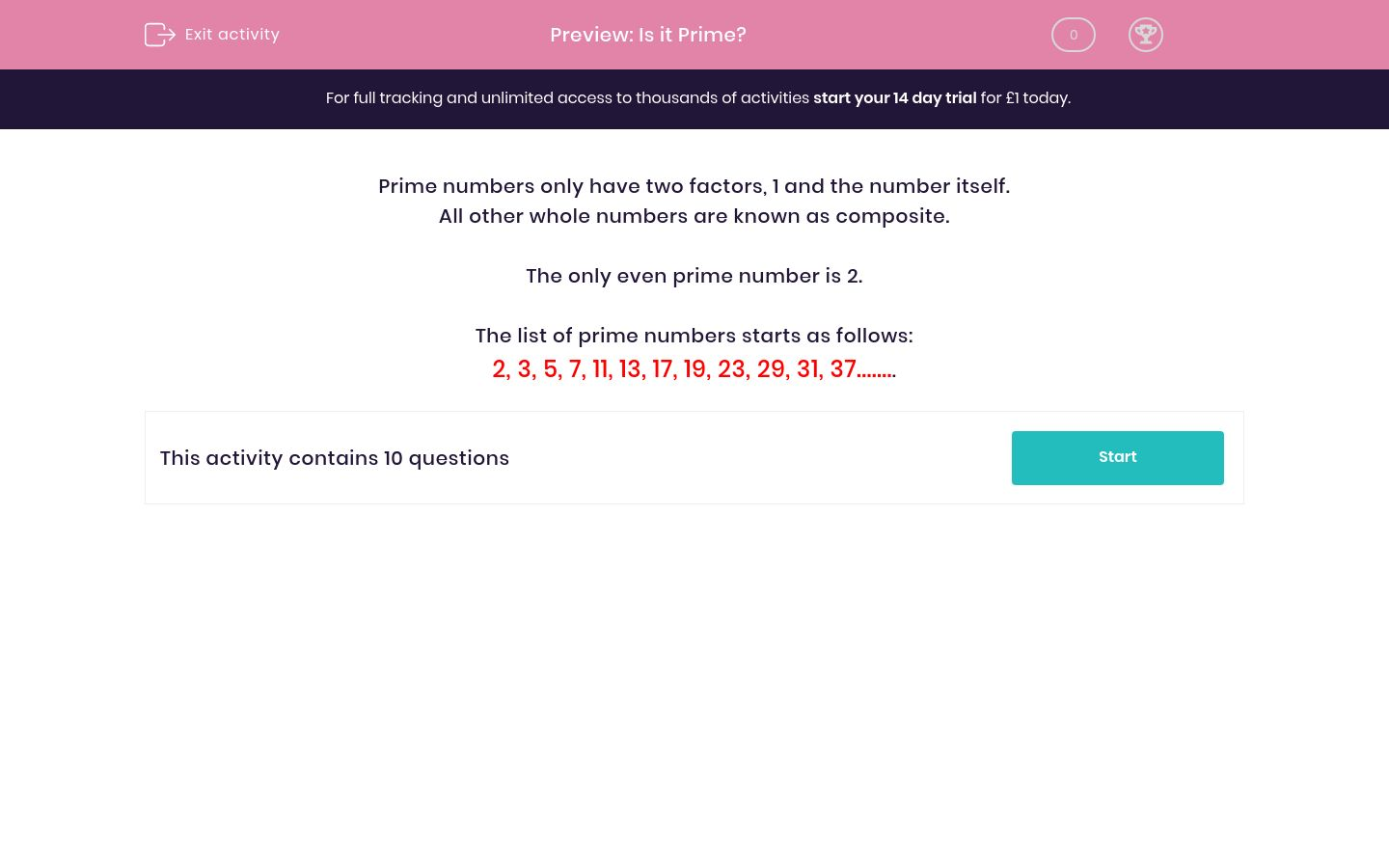# Is it Prime?

In this worksheet, students revise and practise deciding whether or not a given number is prime.Key stage:  KS 2

Curriculum topic:   Maths and Numerical Reasoning

Curriculum subtopic:   Factors and Multiples

Difficulty level:### QUESTION 1 of 10

Prime numbers only have two factors, 1 and the number itself.

All other whole numbers are known as composite.

The only even prime number is 2.

The list of prime numbers starts as follows:

2, 3, 5, 7, 11, 13, 17, 19, 23, 29, 31, 37........

State whether or not the following number is prime:

7

Prime

Not prime

State whether or not the following number is prime:

17

Prime

Not prime

State whether or not the following number is prime:

27

Prime

Not prime

State whether or not the following number is prime:

47

Prime

Not prime

State whether or not the following number is prime:

57

Prime

Not prime

State whether or not the following number is prime:

51

Prime

Not prime

State whether or not the following number is prime:

71

Prime

Not prime

State whether or not the following number is prime:

81

Prime

Not prime

State whether or not the following number is prime:

91

Prime

Not prime

State whether or not the following number is prime:

101

Prime

Not prime

• Question 1

State whether or not the following number is prime:

7

Prime
• Question 2

State whether or not the following number is prime:

17

Prime
• Question 3

State whether or not the following number is prime:

27

Not prime
• Question 4

State whether or not the following number is prime:

47

Prime
• Question 5

State whether or not the following number is prime:

57

Not prime
EDDIE SAYS
57 is divisible by 3.
• Question 6

State whether or not the following number is prime:

51

Not prime
EDDIE SAYS
51 is divisible by 3.
• Question 7

State whether or not the following number is prime:

71

Prime
• Question 8

State whether or not the following number is prime:

81

Not prime
• Question 9

State whether or not the following number is prime:

91

Not prime
EDDIE SAYS
91 is divisible by 7.
• Question 10

State whether or not the following number is prime:

101